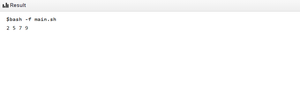Related Articles

# Sorting an Array in Bash using Insertion Sort

• Difficulty Level : Basic
• Last Updated : 20 Jul, 2021

Given an array, arr[] of size N, the task is to sort the array in ascending order using Insertion Sort in bash scripting.

Examples:

Input: arr[] = {9, 7, 2, 5}
Output: 2 5 7 9
Explanation: The array in sorted order is {2, 5, 7, 9}

Input: arr[] = {3, 2, 1}
Output: 1 2 3
Explanation: The array in sorted order is {1, 2, 3}

Approach: Follow the steps below to solve the problem:

• Traverse the given array, arr[] in the range [1, N] and perform the following steps:
• Compare the current element (key) to its predecessor.
• If the key element is smaller than its predecessor, compare it to the elements before. Move the greater elements one position up to make space for the swapped element.
• After completing the above steps, print the sorted array.

Below is the implementation of the above approach:

```# Bash program for the above approach
n = 4
arr = (9 7 2 5)

# Perform insertion sort to sort the array
j = 1
while [ \$j -lt \$n ]
do
c = 0
k = \$(expr \$j - 1)
while [ \$k -ge 0 ]
do
if [ \${arr[k]} -gt \${arr[j]} ]
then
c=\$(expr \$c + 1)
fi
k = \$(expr \$k - 1)
done

x = \$j
y = \$(expr \$j - 1)

while [ \$c -gt 0 ]
do
# Swapping the elements
temp=\${arr[x]}
arr[\$x]=\${arr[y]}
arr[\$y]=\$temp

x=\$(expr \$x - 1)
y=\$(expr \$y - 1)
c=\$(expr \$c - 1)

done

j = \$(expr \$j + 1)
done

# Print the sorted array
echo "\${arr[*]}"
```

Output:

2 5 7 9My Personal Notes arrow_drop_up